This lesson demonstrates the energy exchange between two optical solitons with orthogonal polarizations associated with the orthogonal Raman gain .

Using equations (17a) from the technical description of the Nonlinear dispersive fiber component it can be shown  that the energy exchange between two solitons: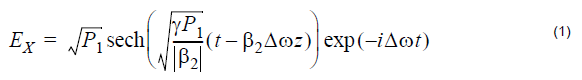where P1 > > P2 and Δ ω > 0 is approximately given by the following expression :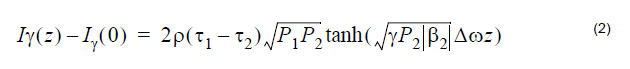Equation 2 denotes the energy of the weaker (low frequency) soliton.

The layout and its global parameters are shown in Figure 1.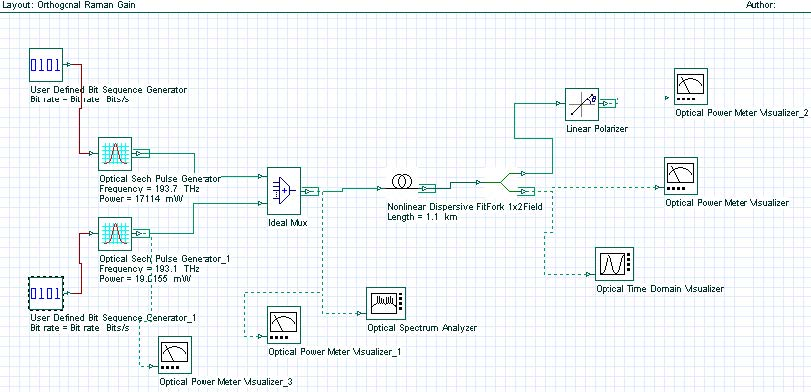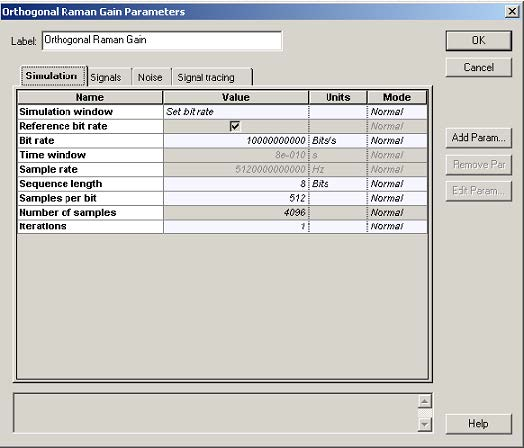Figure 1: System layout and global parameters

Figure 2 gives the setup for the nonlinear dispersive fiber component.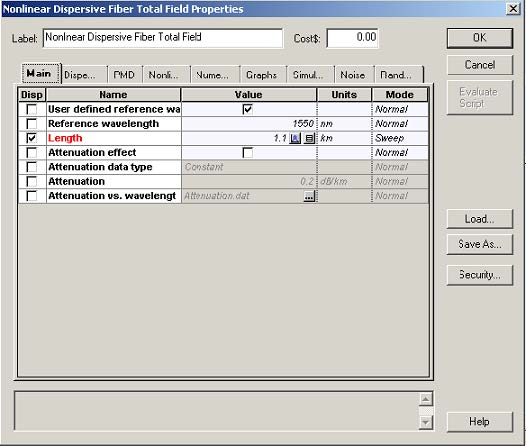Figure 2: Setup parameters

Figure 3 gives the comparison between the analytical result of Equation 2 and the results obtained with OptiSystem.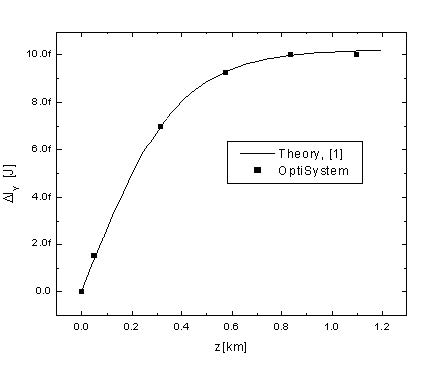Figure 3: Experimental and OptiSystem results

References:
C. R. Menyuk, M. N. Islam and J. P. Gordon, Optics Letters 16 p.566 (1991).